Laws of exponents

Chapter 1 Class 9 Number Systems
Concept wise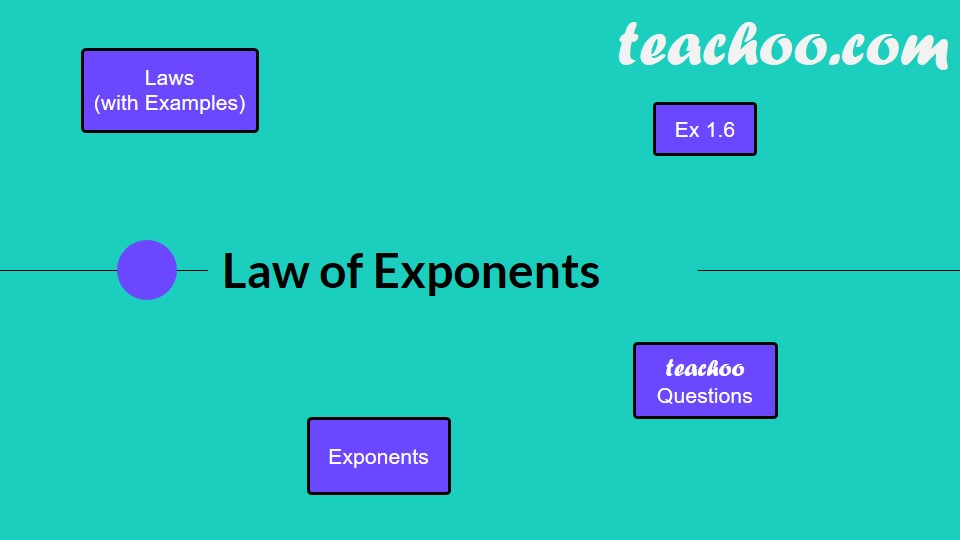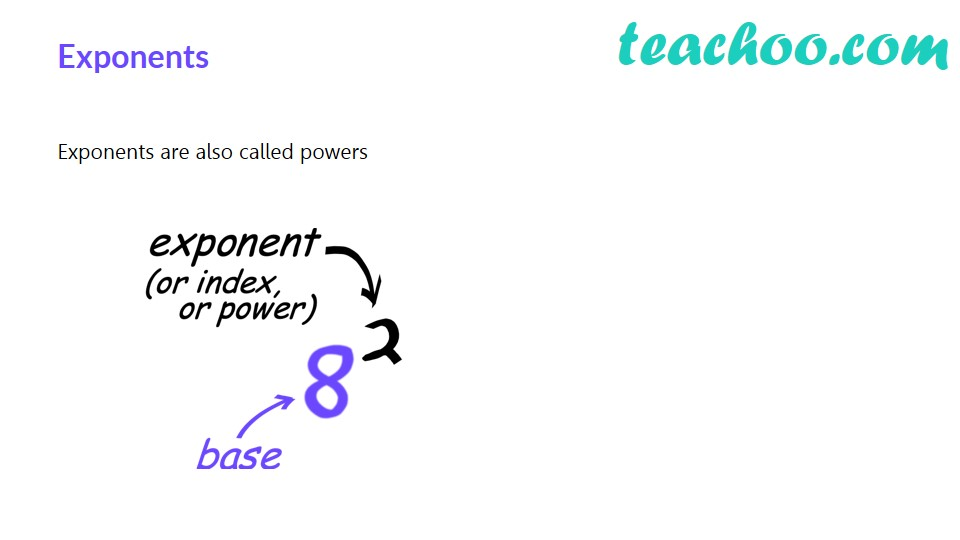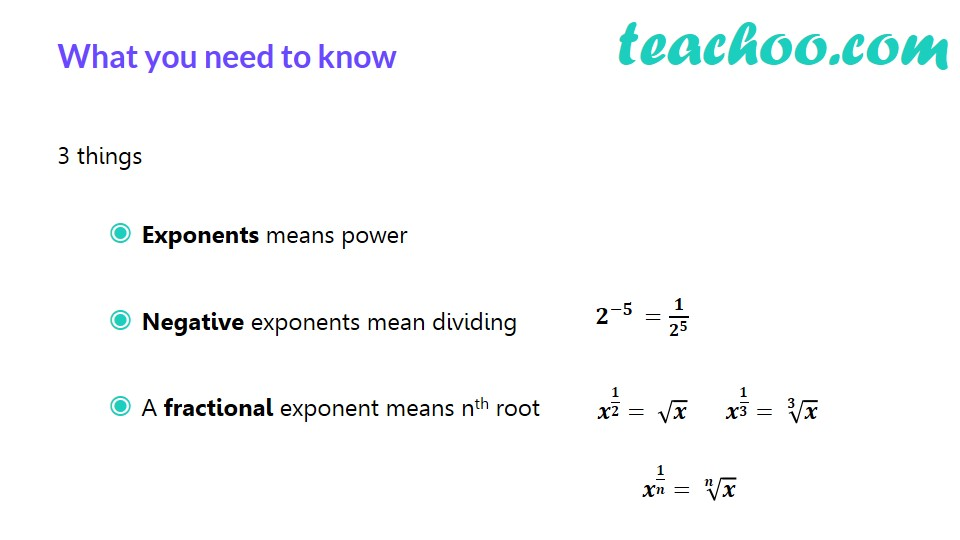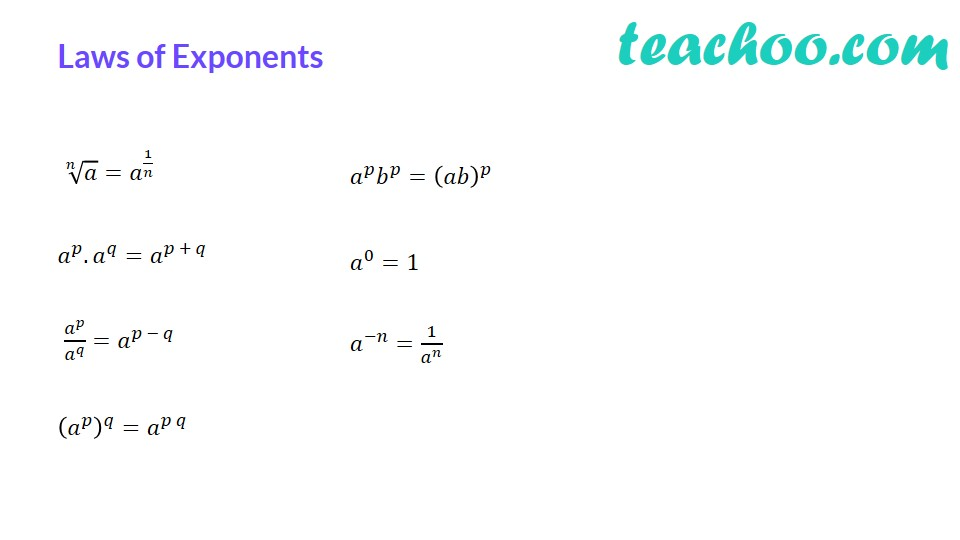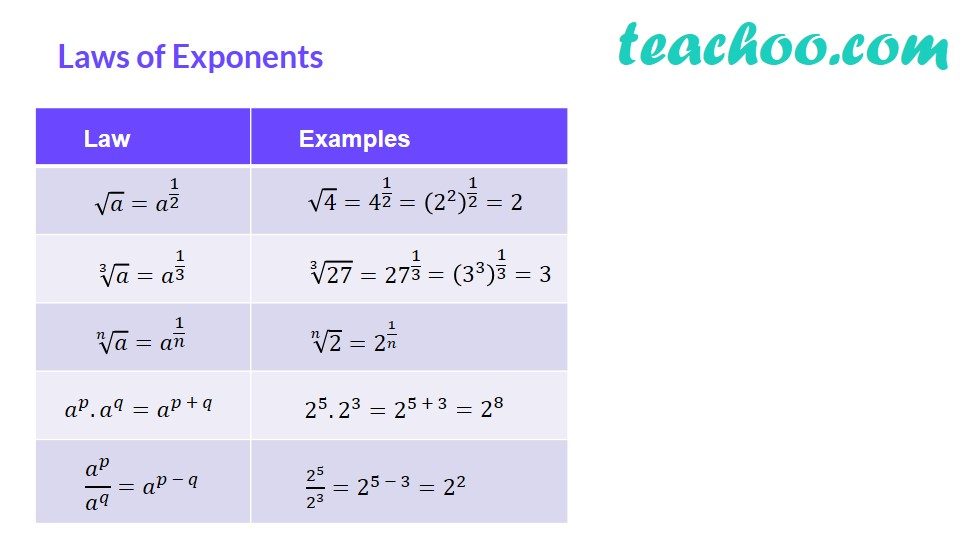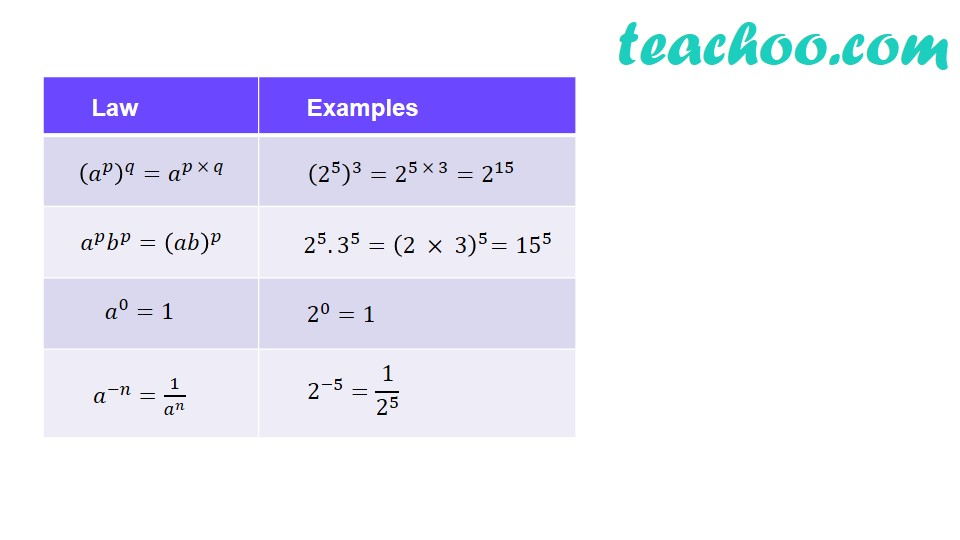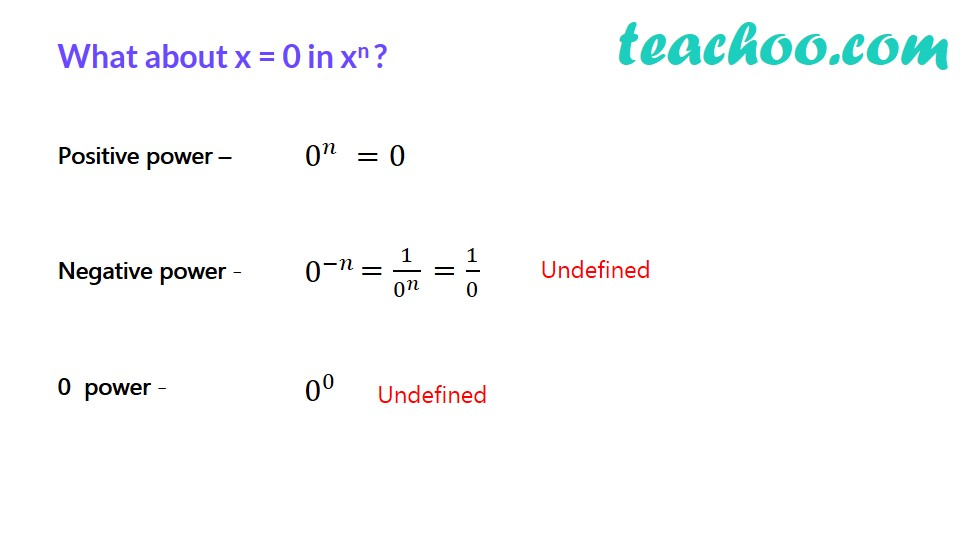Let's first see what exponents are

In 2 5 ,

2 is the base

5 is the exponent (or index)

Let's study the law of indices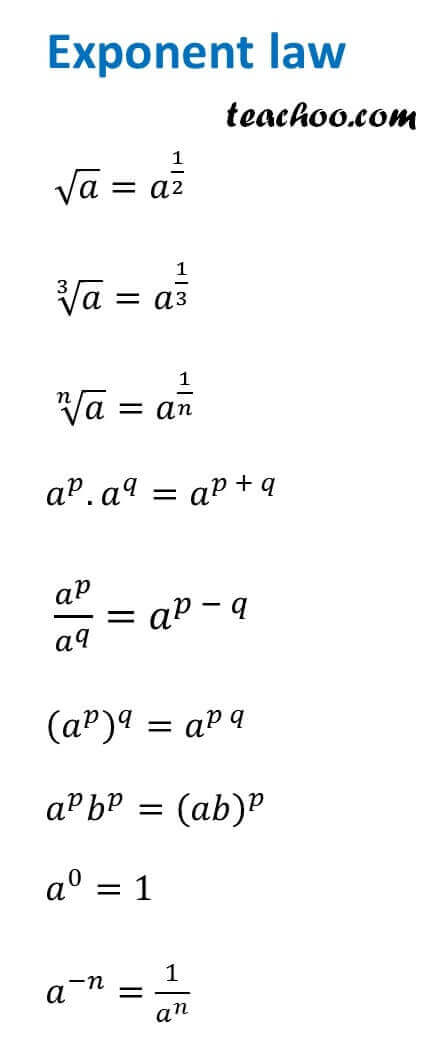• √a = a 1/2

• ∛a = a 1/3

• n √a = a 1/n

• a p.  a q = a p + q
• a p / a q  = a p - q
• a p.  b p = (ab) p
• (a p ) q.  = a pq
• a 0 .  = 1
• a –n.  = 1/a n

## Exponent law with examples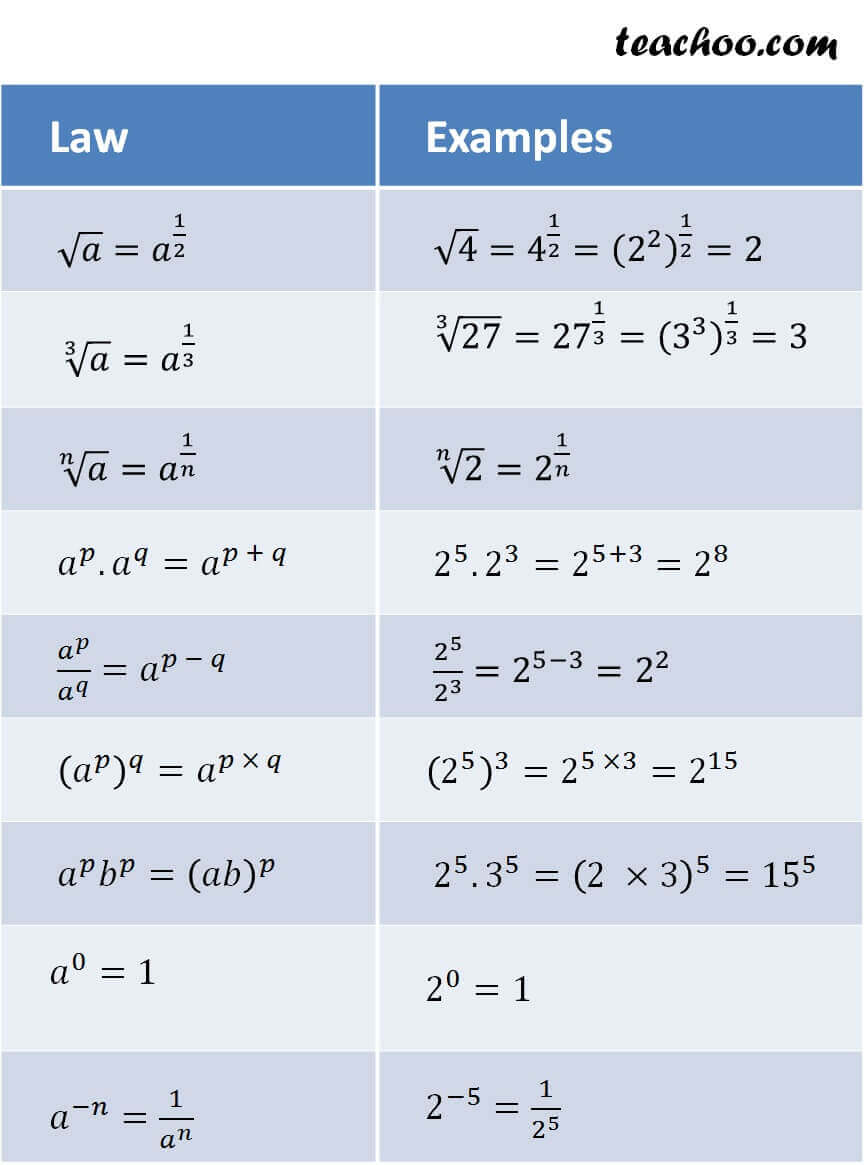Click next for more questions on exponents. You can also check Algebra Formulas

Learn in your speed, with individual attention - Teachoo Maths 1-on-1 Class

### Transcript

Exponents Exponents are also called powers What you need to know 3 things Exponents means power Negative exponents mean dividing A fractional exponent means nth root 𝟐^(−𝟓 )=𝟏/𝟐^𝟓 𝒙^(𝟏/𝟐)= √𝒙 Laws of Exponents √(𝑛&𝑎)=𝑎^(1/𝑛) 𝑎^𝑝.𝑎^𝑞=𝑎^(𝑝 + 𝑞) 𝑎^𝑝/𝑎^𝑞 =𝑎^(𝑝 − 𝑞) (𝑎^𝑝 )^𝑞=𝑎^(𝑝 𝑞) 𝑎^𝑝 𝑏^𝑝=(𝑎𝑏)^𝑝 𝑎^0=1 𝑎^(−𝑛)=1/𝑎^𝑛 Laws of Exponents What about x = 0 in xn ? Positive power – Negative power - 0^𝑛 0 power -